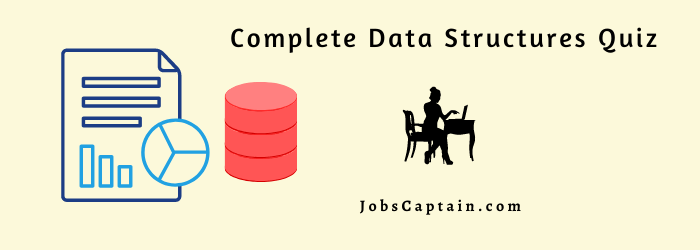# Data Structures MCQ Quiz With Answers – 3## Advance Data Structures MCQ Quiz – 3

Question 1: Matrices with a relatively high proportion of zero entries are called _________ matrices.

(A) sparse

(B) Null

(C) Zero

(D) worse

(A) sparse

Question 2: The Postfix equivalent of the Prefix Notation * + ab – cd is ______________ .

(A) ab + cd – *

(B) abcd +-*

(C) ab+cd*-

(D) ab+-cd*

(A) ab + cd – *

Question 3: Data structure which is capable of expressing more complex relationship than that of physical adjacency is called ______________ .

(A) linear data structure

(C) non linear data Structure

(D) data structure

(C) non linear data Structure

Question 4: A tree is a data structure which represents hierarchical relationship between individual ___________.

(A) data items

(B) fields

(C) nodes

(A) data items

Question 5: In a directed tree any node which has out degree 0 is called a terminal node or ____________.

(A) a tree

(B) a list

(C) a node

(D) a leaf

(D) a leaf

Question 6: In a directed tree if the ordering of the nodes at each level is prescribed then such a tree is called ___________ tree.

(A) directed

(B) structure

(C) ordered

(D) degree of

(C) ordered

Question 7: ______________ a tree means processing it in such a way that each node is visited only once.

(A) Traversing

(B) Implement

(C) Partition

(D) Node

(A) Traversing

Question 8: The length of the path is the number of _____________ on the path.

(A) nodes

(B) fields

(C) data

(D) edges

(D) edges

Question 9: The children node of same parent is called ____________.

(A) binary tree

(B) tree

(C) sibling

(D) list

(C) sibling

Question 10: The situation in linked list START = NULL is called _________.

(A) Overflow

(B) Underflow

(C) Zero

(D) None of the above

(B) Underflow

Question 11: A code which deals about short form of a program is called __________ code.

(A) program

(B) data

(C) pseudo

(D) derived

(C) pseudo

Question 12: Which of the application may use a stack?

(A) Expression Evaluation

(B) Keeping track of local variables at run time.

(C) Syntax analyzer for a compiler

(D) All of the above.

(A) Expression Evaluation

Question 13: The queue which wraps around upon reaching the end of the array is called as____________.

(A) circular queue

(D) representation of queue

(A) circular queue

Question 14: A ______________ is a reference to a memory location, which is used to store data that is described in a data type.

(A) element

(B) variable

(C) pointer

(D) memory

(B) variable

Question 15: If the elements A, B, C and D are placed in a stack and are deleted one at a time, what is the order of removal?

(A) ABCD

(B) DCBA

(C) DCAB

(D) ABDC

(B) DCBA

Question 16: ____________ has certain attributes or properties which may be assigned values.

(A) field system

(B) record

(C) entity

(D) files

(C) entity

Question 17: ________ is the situation where data-structure is empty.

(A) Overflow

(B) Underflow

(C) Null

(D) Empty

(B) Underflow

Question 18: When elements are deleted the nodes go to ___________.

(A) registers

(B) free pool

(C) recycle bin

(D) gets deleted permanently

(B) free pool

Question 19: Expression into postfix expression: (A – B) * (D / E)

(A) ABDE – * /

(B) – * / ABDE

(C) A B – D E * /

(D) * – A B / D E

(D) * – A B / D E

Question 20: Each data item in a record may be a group item composed of sub-items; those items which are indecomposable are called __________ .

(A) elementary items

(B) atoms

(C) scalars

(D) structure

(D) structure

Question 21: Quick sort uses __________ for implementation.

(A) recursion

(B) traversal

(C) heaps

(D) queues

(A) recursion

Question 22: Program module contains its own list of variables called ____________.

(A) global

(B) scope

(C) local

(D) external

(C) local

Question 23: The string with zero characters is called _____________.

(A) null string

(B) zero string

(C) one string

(D) empty string

(D) empty string

Question 24: In variable length storage two dollar signs are used to signal the __________.

(A) end of the string

(B) beginning of the string

(C) mid-level of the string

(D) index

(A) end of the string

Question 25: Quotation marks are also called as ____________.

(A) string delimiters

(B) period

(C) stopper

(D) string

(A) string delimiters

Question 26: A string ‘s’ consists of x, y and if x is an empty string then y is called as ___________.

(A) initial substring

(B) substring of s

(C) node of the string

(D) index

(A) initial substring

Question 27: Who invented Quick sort procedure?

(A) Hoare

(B) Sedgewick

(C) Mellroy

(D) Coreman

(A) Hoare

Question 28: For the heap sort, access to nodes involves simple _______________ operations.

(A) binary

(B) arithmetic

(C) algebraic

(D) logarithmic

(B) arithmetic

Question 29: What is an external sorting algorithm?

(A) Algorithm that uses tape or disk during the sort

(B) Algorithm that uses main memory during the sort

(C) Algorithm that involves swapping

(D) Algorithm that are considered in place

(A) Algorithm that uses tape or disk during the sort

Question 30: Allocating memory for arrays during program compilation is ___________.

(A) dynamic memory allocation

(B) memory allocation

(C) static allocation

(D) random allocation